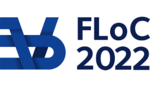FLOC 2022: FEDERATED LOGIC CONFERENCE 2022
TALK KEYWORD INDEX

A
abstract rewriting
AC-Unification
Agda
Algebra Interpretations
anti-unification
Artin gluing
Automatic Complexity Analysis
axiom of choice
B
Backreferences
Bicategories
C
Call-by-name
call-by-push-value
Call-by-value
canonicity
Categorical models
categorical semantics
Certified Algorithms
Choice sequences
Church's thesis
Circular proofs
Classical Logic
Combination Problem
combinatorial proofs
combinatory logic
computability theory
computational effect
concurrency
confluence
constructive mathematics
constructive type theory
Coq
counter automata
Curry-Howard correspondence
Cut Elimination
D
decidability
decreasing diagrams
Dedukti
deep inference
Denotational semantics
Dependency Pairs
dependent type theory
Diagrammatic language
Display calculus
distributive laws
division
E
emptiness
evaluation order
Extensional Type Theory
F
fibrations
finiteness
first-order logic
fixed points
formal methods
futures
G
generalisation
Generalized applications
generic effects
gluing
Guarded Recursion
H
higher-order deduction
higher-order term rewriting
homotopy type theory
I
Implicit Computational Complexity
infinite proofs
information flow
Interactive Theorem Proving
Intuitionism
intuitionistic logic
L
lambda calculus
lambda-calculus
Law of Excluded Middle
linear logic
lob induction
logical framework
logical frameworks
logical relations
M
Matching
matching modulo AC
mechanization
Memory automata
metric spaces
modal type theory
modularity
monoidal closed categories
N
Nested sequent calculus
nominal algorithms
noninterference
normalization
O
operational semantics
P
Peano arithmetic
phase distinction
Polynomial functors
polynomial termination
probabilistic lambda-calculus
probabilistic rewriting
program distance
Program Verification
proof nets
proof theory
Pure Type Systems
PVS
Q
quantitative algebras
Quantitative types
Quantum Computation
Quantum Computing
R
Realizability
References
Regular expressions
Regular Theories
rewriting
rule formats
S
Sequent Calculus
sequentiality
series-parallel orders
set theory
sized types
Solvability
Species of structures
Static Program Analysis
strategies
string diagrams
Structural operational semantics
synthetic computability
Synthetic domain theory
synthetic Tait computability
T
Tennenbaum's theorem
term rewriting
Termination
theorem proving
topos theory
tree automata
tree grammar
type theory
type universes
type-based termination
U
Undecidability
Z
ZX-calculus